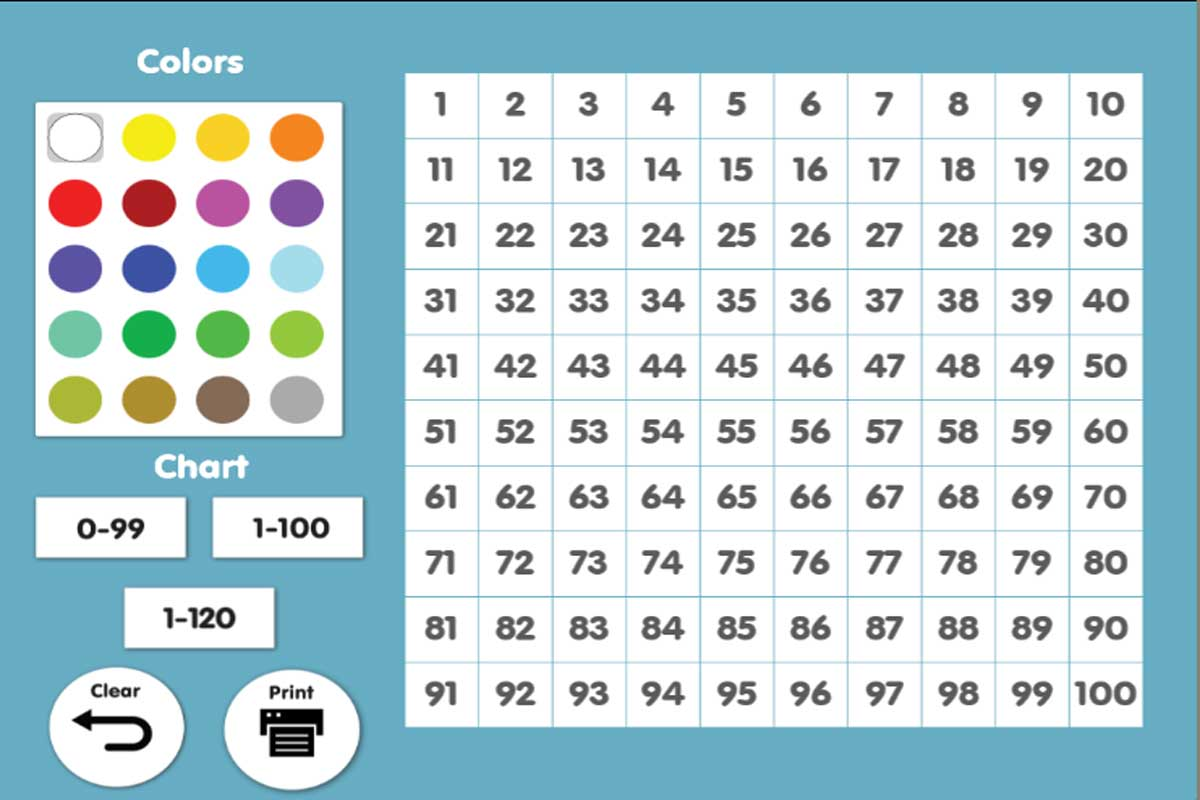# Interactive Number Chart {Free}Here is a great find at ABCYa.com: Interactive Number Chart. Hundred charts provide an excellent, visual way to become familiar with numbers.

The hundred chart…is one of the most flexible and useful devices you will ever find for helping your child to understand numbers and the basic arithmetic processes. Use it almost every day in your teaching.

Ruth Beechick, An Easy Start in Arithmetic

The number chart at ABCYa allows you to use different colors to create your chart, and then print a copy.

There are many ways to use a hundred number chart:

• Skip count by 10s, 5s, 2s, or 3s by coloring in the appropriate corresponding squares.
• Use the chart for simple addition; for example, when calculating 9+3=, add 3 to 9 by finding 9 and counting forward 3 squares.
• Count backward by 10s, 5s, or 2s.
• Learn simple subtraction; for example, when calculating 9-3=, begin at 9 and count backward 3 squares.
• Look for patterns. For example, try adding 9 to any number.
• Play a game (see resources below).
• Find the number. Think of a number and as in 20 questions, have the student ask questions to identify the number.
• Generate a list of prime numbers by using the Sieve of Eratosthenes.

The options are nearly endless.

Enjoy this free interactive!

An Easy Start in Arithmetic by Ruth Beechick